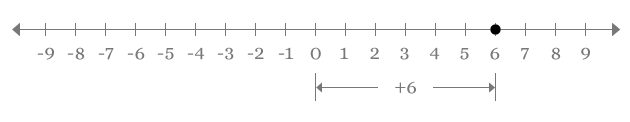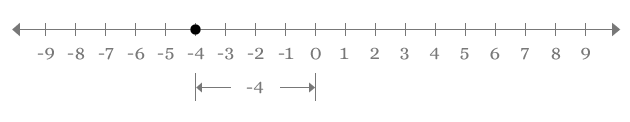# Number line

Displaying numbers with equidistant separation on a straight line is defined as number line.

Number Line is a straight line, consists of real numbers which are separated by equal distance. It is useful to identify the location of a point on a plane and also useful to know the distance of a point from a known point.

## Drawing Number LineA number line is constructed from a straight line and it is usually displayed in horizontal direction.Divide the straight line into two equal parts and assume the middle point of the straight line is denoted by zero $\left(0\right)$ number. It is called origin of the number line.Divide each part into numerous equal divisions. Each division is having same length. Denote divisions of the right side part with positive real numbers and also denote divisions of the left side part with negative real numbers. Actually, integers are used to denote length of the division in the number line because all integers are real numbers.

The representation of the number line is in one direction and the concept of the number line developed from Cartesian coordinate system. Therefore, number line is known as one dimension form of Cartesian coordinate system and it is also called as one dimensional space.

### Identifying a Point

One dimensional space is used to identify the location of any point.For example, the position of a point is $6$. There is no sign for the number. So, it should be considered as positive number. The point has to be marked in right side direction at $6$ units from the origin and it is denoted by a dot symbol at $6$ on the number line.Consider another point and assume the location of the point is $–4$. The number has negative sign. So, it has to be marked on number in left side direction at $4$ units distance from the origin.